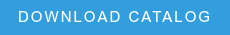One common technique applied in microscopic image analysis is colocalization, which is the quantification of the spatial relationship between two or more elements in color or multichannel image(s)[i]. Qualitatively, it is the degree of spatial overlap of multiple signals coming from the same region of interest. This is useful for a number of applications in biology where fluorescent-labeling and light microscopy are used as the principle means to measure the presence of a particular biomarker of interest. Whether it be restricted to the subcellular compartmentalization of certain proteins, or the more macroscopic morphology of tissue components such as vasculature, colocalization is a critical tool in the arsenal of quantitative techniques for image-based data analysis.

In practice, colocalization is normally performed by correlating the intensity value of each pixel between two or more channels[ii]. This is known as pixel intensity spatial correlation, and it is a statistical-based approach to colocalization. It can be calculated in several ways, but the most common two metrics are the Pearson correlation coefficient, and the Manders’ split coefficients. These parameters measure the degree to which signal from two channels is linearly correlated to one another and gives a value between -1 and 1 for Pearson’s, and between 0 and 1 for Manders. The value of 0 means no correlation, 1 (and -1) means perfect correlation (or anti-correlation). Another common approach is to perform object thresholding and segmentation to colocalize two elements based upon there coordinates. This is a much more robust method for colocalization, but requires sufficient image quality to perform good segmentation. An example of a series of image with overall moderate colocalization is given below. The Pearson’s correlation coefficient for the stack is 0.74.

[i] Dunn, K.W., Kamocka, M.M., McDonald, J.H., A practical guide to evaluating colocalization in biological microscopy. (2011). Am. J. Physiol. Cell Physiol. 300(4): C723-C742.

[ii] Bolte, S., Cordelieres, F.P., Aguided tour into subcellular colocalization analysis in light microscopy. (2006). Journal of Microscopy 224(3): 213-232.